## Thursday, 31 October 2013

### RAII is a must know technique for c++ programmers, no excuse.

RAII(resource acquisition is initialization), in a nutshell, is same as "let the object handle the resource".I use the word resource but not memory, because RAII can guard more than memory(mutex, db connnection, socket, file and every resource you can think of).There are many blogs, famous technical forum, gurus suggestion, mention and emphasize how important and what RAII is.

C++ is a big, complexity language, I have no idea I need to take how many years to learn every corners of this powerful beast, but we do not need to learn all of it. We only need to know the most essential part of c++, RAII is one of them.You may not use any TMP(template meta programming) , multi inheritance(please think carefully before you use this feature), design any allocators, don't know what is policy based design, have no idea how type_traits work, do not familiar with operator overloading, but you will always find out that RAII could help you write good codes, make your codes become much more easier to maintain, read and more robust.

If you don't believe it, let us look at a simple example.

```#include <iostream>
#include <new>

int main()
{
int *arr = nullptr;
int *max = nullptr;
double *double_arr = nullptr;

try{
arr = new int;
max = new int(300);
double_arr = new double;

//.....do something......

//handle your garbages if something happen
goto cleanUp;
//......
std::cerr<<ex.what()<<std::endl;
}

cleanUp:
if(arr){
delete []arr;
arr = nullptr;
}
if(max){
delete max;
max = nullptr;
}
if(double_arr){
delete []double_arr;
double_arr = nullptr;
}
}
```

What is the problem with this example?They are perfectly legal and safe in C++?If you don't get it, let me ask you a problem.

Q : What if we need to handle more resource?

The suck codes proposed by the naive answer may look like

```#include <iostream>
#include <new>

int main()
{
int *arr = nullptr;
int *max = nullptr;
double *double_arr = nullptr;
float *float_max = nullptr;
float *float_arr = nullptr;

try{
arr = new int;
max = new int(300);
double_arr = new double;
float_max = new float(3.14);
float_arr = new float;

//.....do something......

//handle your garbages if something happen
goto cleanUp;
//......
std::cerr<<ex.what()<<std::endl;
}

cleanUp:
if(arr){
delete []arr;
arr = nullptr;
}
if(max){
delete max;
max = nullptr;
}
if(double_arr){
delete []double_arr;
double_arr = nullptr;
}
if(float_max){
delete float_max;
float_max = nullptr;
}
if(float_arr){
delete []float_arr;
float_arr = nullptr;
}
}
```

You will have to alter two places whenever you need to add or delete the resources.This kind of c style codes are error prone, hard to maintain and unbearable, they are almost as worse as(even worse than it if we have to take care of exception) the old school c codes which heavily depends on goto to clean up resources, this kind of codes will become unreadable in short times. I believe this is one of the reason why Bjarne said "Aim to write idiomatic C++: avoid simply writing code in the style of your previous language using C++ syntax; there is little to be gained from simply changing syntax.".For any C++ programmers who know how important and powerful RAII is, they can't bear this kind of horrible codes.

How could RAII help us?

```#include <iostream>
#include <memory>
#include <new>
#include <vector>

int main()
{
try{
std::vector<int> arr(100);
std::unique_ptr<int> max(new int(300));
std::vector<double> double_arr(300);
std::unique_ptr<double> double_max(new double(300));
std::vector<float> float_arr(100);
std::unique_ptr<float> float_max(new float(100));

//...do something....

//the object will release the resources automatically
//even the exceptions thrown

std::cerr<<ex.what()<<std::endl;
}
}
```

Do you see that?The codes become much more easier to read, because we don't need to release the resource manually, we don't need to change the codes in two places.The codes become more robust, because we will not forget to release the resources again.More than that, it is exception safe too.c++ is good because you can deal with pointer and memory management, in c you have to deal with pointer and memory management.

There are many kinds of coding standards, rules in many teams, not all coding standards suit for all teams and all applications.Whatever it is, RAII is a must know technique and should be obey when you can. If you are using delete in your codes, maybe you are doing something wrong or writing worse codes in C++, even you need to use delete(building some infrastructures, like smart pointer, containers and so on), do remember to protect it by object, always remember to follow the rule of RAII.Pointer is good at access data, but not resource management, leave your resource to class(RAII).

In my humble opinion, if a C++ programmer don't know the concepts of RAII, he/she will generate lower quality, worse performance, less reliable codes most of the times(just like the suck codes show by the examples).  It is always a good question to test your interviewers, ask them they notice how important RAII in C++ or not.If they don't know it, don't hire them(or teach them if you think the candidates are worth to).If you were an interviewer and find out the company don't take RAII seriously, then you should be careful.

## Wednesday, 30 October 2013

### openCV and artificial neural network(ANN)--01 : simple OCR engine with openCV2

To get a better image of how to make use of the artificial neural network provided by openCV, I decided to do develop a simple OCR(optical character recognition) engine by CvANN_MLP.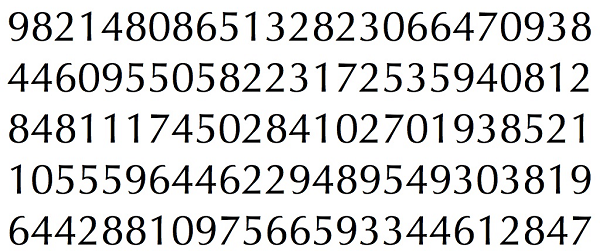graph_00(digits for training)graph_01(digits for classify)

Step 1 : segment the digits of graph_00

```std::string const prefix("/Users/Qt/program/blogsCodes/");
cv::Mat img = cv::imread(prefix + "pic/digits00.png");
if(img.empty()){
return - 1;
}

detectCharacter dc;
std::vector training_data = dc.segment(img, {10, 10});
```

implementation of segment

```std::vector detectCharacter::segment(cv::Mat const &input,
cv::Size const &crop_size)
{
CV_Assert(input.type() == CV_8UC3);

//step 1 : binarize the image
cv::cvtColor(input, gray, CV_BGR2GRAY);
cv::GaussianBlur(gray, gray, cv::Size(5, 5), 0);

cv::Mat binary = gray;
cv::threshold(gray, binary, 0, 255,
cv::THRESH_BINARY_INV + cv::THRESH_OTSU);

//step 2 : find the contours
cv::findContours(binary.clone(), contours, CV_RETR_EXTERNAL,
CV_CHAIN_APPROX_SIMPLE);

//step 3 : get the bounding rect of each contours
for(auto &data : contours){
min_rects.emplace_back(cv::boundingRect(data));
}

//step 4 : crop each digits into the vector
std::vector<cv::Mat> digits;
for(size_t i = 0, size = contours.size(); i != size; ++i){
cv::Mat const digit = crop_digit(binary, min_rects[i],
contours[i], crop_size);
digits.emplace_back(digit);
}

return digits;
}
```

implementation of crop_digit

```/**
* @brief crop a character in the input
* @param input : input image
* @param rect  : location of the character
* @param points : contours
* @param size : size of the result
* @return character after crop
*/
cv::Mat detectCharacter::crop_digit(cv::Mat const &input,
cv::Rect const &rect,
std::vector<cv::Point> const &contour,
cv::Size const &size)
{
digit.setTo(0);

auto digit_ptr = digit.ptr<uchar>(0);
for(int row = 0; row != mask.rows; ++row){
for(int col = 0; col != mask.cols; ++col){
//only take the pixels equal to 255 and
//lie in the region surrounded by the contour
cv::pointPolygonTest(contour,
{col + rect.x, row + rect.y},
false) >= 0){
*digit_ptr = 255;
}
}
}

cv::resize(digit, digit, size);

return digit;
}
```

Before I return the digit, I resize the digit into a specific size, because the samples of the ANN have to be same size and same type.

Step 2 : set up training labels

This step is very verbose, I have to map the number of the digits after segmentation to appropriate value.Since there are 125 digits after segmentation, it took me some times to make it done.

```std::vector<int> const training_labels
{
7, 4, 8, 2, 1, 6, 4, 4, 3, 3, 9, 5, 6, 6, 5,
7, 9, 0, 1, 8, 8, 2, 4, 4, 6, 9, 1, 8, 3, 0,
3, 9, 4, 5, 9, 8, 4, 9, 2, 2, 6, 4, 4, 6, 9,
5, 5, 5, 0, 1, 1, 2, 5, 8, 3, 9, 1, 0, 7, 2,
0, 1, 4, 8, 2, 0, 5, 4, 7, 1, 1, 1, 8, 4, 8,
2, 1, 8, 0, 4, 9, 5, 3, 5, 2, 7, 1, 3, 2, 2,
8, 5, 0, 5, 5, 9, 0, 6, 4, 4, 8, 3, 9, 0, 7,
4, 6, 6, 0, 3, 2, 8, 2, 3, 1, 5, 6, 8, 0, 8,
4, 1, 2, 8, 9
};
```

Step 3 : write the training data and training labels into xml file

We could transform the data and labels to suitable form without writing them to xml too, we don't have any obligation to write the data into xml, write it or not is depend on your needs.

```void write_digits_xml(std::vector<cv::Mat> &training_data,
std::vector<int> const &training_labels)
{
cv::Mat train_data;
cv::Mat train_labels;

for(auto &data : training_data){
//same as data.reshape(1, 1),
//data.convertTo(data, CV_32F);
OCV::transform_to_svm_training_data(data);
train_data.push_back(data);
}

cv::Mat(training_labels).copyTo(train_labels);

cv::FileStorage fs("digits.xml", cv::FileStorage::WRITE);
fs << "TrainingData10x10" << train_data;
fs << "Labels" << train_labels;
}
```

Step 4 : Segment the digit of the graph_01(target)

```cv::Mat target = cv::imread(prefix + "pic/digitTarget00.png");
if(target.empty()){
return -1;
}

std::vector<cv::Mat> target_digits = dc.segment(target, {10, 10});
std::vector<cv::Mat> temp_target_digits; //for verification
for(auto &data : target_digits){
temp_target_digits.emplace_back(data.clone());
OCV::transform_to_svm_training_data(data);
}
```

At step 4,  I segment the digits from graph_01 and create one more buffer--temp_target_digits to store the digits after segmentation, because the target_digits have to transform to one row and one channel matrix, so we need a buffer to retain the digit after segmentation for verification on the next step.

Step 5 : train and classify the digit

```characterRecognizer cr(prefix +
"simpleOCR/build-exercise00-Qt5_1_1_clang_3_2-Release/digits.xml");
cr.train(10, 10);

for(size_t i = 0, size = target_digits.size(); i != size; ++i){
int const classify = cr.classify(target_digits[i]);
std::cout<<classify<<std::endl;
cv::imshow("", temp_target_digits[i]);
cv::waitKey();
}
```

At step 5, I train the neural network, classify the image after segmentation(we need to transform the image want to classify to one row, one channel and CV_32F matrix), and verify the result correct or by comparing the digits after segmentation and the result of classify.

implementation of train

```/**
* @brief overload of train, you can specity the training
*        data and training labels by this overload function
* @param train_data : the training data
* @param labels : the training labels
* @param nlayers : layers of the network
* @param num_char : number of the characters,
* it is equal to the type of the training labels(training classes)
*/
void characterRecognizer::train(cv::Mat const &train_data,
cv::Mat const &labels, int nlayers,
int num_char)
{
CV_Assert(!train_data.empty() && !labels.empty());

num_charecter = num_char;
int buffer[] = {train_data.cols, nlayers, num_char};
cv::Mat const layers(1, 3, CV_32S, buffer);
ann.create(layers, CvANN_MLP::SIGMOID_SYM, 1, 1);

//Prepare trainClases
//Create a mat with n trained data by m classes
cv:: Mat train_classes;
train_classes.create(train_data.rows, num_char, CV_32F);
for( int i = 0; i !=  train_classes.rows; ++i){
int const label = *labels.ptr<int>(i);
auto train_ptr = train_classes.ptr<float>(i);
for(int k = 0; k != train_classes.cols; ++k){
*train_ptr = k != label ? 0 : 1;
++train_ptr;
}
}

cv::Mat const weights = cv::Mat::ones(1, train_data.rows, CV_32FC1);

//Learn classifier
ann.train(train_data, train_classes, weights);
}
```

The principle of this method could found at this link. In this example I treat all of the region of the segmented digit as features, exactly this is not an optimal solution, there are many ways to extract the features of the segmented digit.

Although the hit rate is high, from the view of machine learning, this method is far from perfect, because it may overfitting the training set, there are many algorithms to help us verify that it is underfitting, overfitting and give us some hints about how to gain a better training result. Don't jump to the conclusion that adding more training examples can improve the result, collect those examples take a lot of times, use some techniques(learning curve, bias, variance, model selection and so on) to verify how should you improve the result before you decide to collect more training examples. This is same as the performance issues of programming, don't guess, measure.

## Monday, 28 October 2013

### openCV and artificial neural network(ANN)--00 : How to construct the labels of ANN

openCV2 implement multi-layer perceptrons(MLP), we could use it by declare CvANN_MLP. To train the classifier of the ANN, we need to create training data and labels as we did in the SVM, but the training labels are a bit different.Instead of an Nx1 matrix where N stand for the samples and 1 present the classes.The labels of an ANN are NxM, the N is the same as SVM(sample numbers), M is a classes which set 1 in a position(i, j) if the row i is classified with column j.

The question is, how do we feed the ANN with proper data format?The document do not mention about this, but thanks to the book and the forum, this question is solved(at-least I think so).

The way of creating the xml is same as the SVM.But we need some post processing to convert the labels into a two dimensions array before we pass the labels into the function train().

This example intent to do a simple OCR training with numeric alpha(an examples from the ch5 of the book with some simplification).

First, we write the data of the samples and associative labels to the cv::Mat.

```//for the sake of simplicity, I neglect some errors checking

#include <opencv2/imgproc/imgproc.hpp>
#include <opencv2/highgui/highgui.hpp>

#include <iostream>

int main ( int argc, char** argv )
{
char const *path = argv;

cv::Mat trainingData;
//35 means there are 35 samples for '0';
//40 means there are 40 samples for '1' and so on
int const numFilesChars[]={35, 40, 42, 41, 42,
30, 31, 49, 44, 30,
};
char const strCharecters[] = {'0','1','2','3','4',
'5','6','7','8','9'};

cv::Mat trainingLabels(0, 0, CV_32S);
int const numCharacters = 10;
//this part is same as the SVM
for(int i = 0; i != numCharacters; ++i){
int numFiles = numFilesChars[i];
for(int j = 0; j != numFiles; ++j){
std::cout << "Character "<< strCharacters[i] <<
" file: " << j << "\n";
std::stringstream ss;
ss << path << strCharacters[i] << "/" << j << ".jpg";
//assume the img is always continuous
img.reshape(1, 1);
trainingData.push_back(img);
trainingLabels.push_back(i);
}
}

trainingData.convertTo(trainingData, CV_32FC1);

cv::FileStorage fs("OCR.xml", FileStorage::WRITE);
fs << "TrainingData" << trainingData;
fs << "classes" << trainingLabels;

return 0;
}
```

second step is read the data and convert the trainingLabels to a proper format of the CvANN_MLP asked for.

```cv::FileStorage fs;
CV::Mat trainData;
Mat classes;
fs["TrainingData"] >> trainData;
fs["classes"] >> classes;

//the 1st element is the input layer(the features of the samples)
//the last one is the outpt layer(how many kinds of data you
//want to classify?)
//all inbetween are for the hidden ones.
int buffer[] = {trainData.cols, nlayers, numCharacters};
//you could specify the layers as 3 rows, 1 column too
cv::Mat const layers(1, 3, CV_32SC1, buffer);
ann.create(layers, CvANN_MLP::SIGMOID_SYM, 1, 1);

//Prepare trainClases
//Create a mat with n trained data by m classes
cv:: Mat trainClasses(trainData.rows, numCharacters, CV_32FC1);
for( int i = 0; i !=  trainClasses.rows; ++i){
int const labels = *classes.ptr<int>(i);
auto train_ptr = trainClasses.ptr<float>(i);
for(int k = 0; k != trainClasses.cols; ++k){
*train_ptr = k != labels ? 0 : 1;
++train_ptr;
}
}

cv::Mat const weights = cv::Mat::ones(1, trainData.rows, CV_32FC1);

//Learn classifier
ann.train(trainData, trainClasses, weights);

```

In the first step, we load the labels as a Nx1 matrix, but in the second step, we need to "unfold" it to NxM matrix( trainClasses).That is what the loop are doing about.N equal to the number of samples and M equal to the number of the data you want to classify.

Ex :
the first sample is '0', so the labels will map to
1 0 0 0 0 0 0 0 0 0
the second sample is '1', so the labels will map to
0 1 0 0 0 0 0 0 0 0
ans so on

## Saturday, 26 October 2013

### openCV : Construct the images suitable for SVM

The example from document show us some basic about the SVM, but it don't tell us how to incorporate with image. I had a hard time to figure out how to do it correctly until I study the boo--mastering openCV with practical computer vision projects.

In a nutshell, the data of SVM needed are training data and labels.

Training data

rows :  corresponding to specific samples(ex : images)

columns :  corresponding to the features(ex : pixels)

In the case of image, all of the pixels values would be considered as features.

```cv::Mat trainingData;
for(size_t i = 0; i != numTrainData; ++i){
std::stringstream name;
name<<leaf<<i<<".png";
//reshape it to 1 channel and 1 row
samples.reshape(1, 1);
trainingData.push_back(samples);
}
//convert all of the image to floating point values
trainingData.convertTo(trainingData, CV_32F);
```

That is, if the original samples are look like a 4X4, after transform, it would become a 1X16 matrix.

Although this is an example for image, but it could apply to everything, the most important part is transform the samples into  a 1 row and 1 channel cv::Mat, put all of the features(data) of the samples into the column.

The function of reshape is very convenient, but it could not tackle with non-continuous image, we have to transform them into a suitable cv::Mat by ourselves.Here is a generic transform function design for images.

```template<typename T = uchar, typename U = float>
void transform_to_svm_training_data(cv::Mat &input)
{
if(input.isContinuous()){
input = input.reshape(1, 1);
input.convertTo(input, cv::DataType<U>().depth);
return;
}

cv::Mat output(1, input.total() * input.channels(),
cv::DataType<U>().depth);
auto output_ptr = output.ptr<U>(0);
OCV::for_each_channels<T>(input, [&](T a)
{
*output_ptr = a;
++output_ptr;
});

input = output;

}
```

There are one more thing to keep in mind, all of the samples of the training data should have same numbers of features, all of the features should have the same type.The codes of OCV::for_each_channels can found on github, the principal of it could refer to opencv and generic programming.

Labels

rows : same as the number of rows of the training data, the samples and the rows of the labels are mapping to each other.

columns : specify what are the samples belong to? For example if you were classifying leafs and non- leafts, you would need to specify which one is leaf and which one is non-leaf in the training data(ex : 1 == leaf; -1 == non-leaf).

Examples

```cv::Mat trainingImages;
std::vector<int> trainingLabels;
for(int i = 0; i != numPlates; ++i)
{
std::stringstream ss;
ss << path_Plates << i << ".jpg";
transform_to_svm_training_data(img);
//every labels are associated to the training data
trainingImages.push_back(img);
trainingLabels.emplace_back(1);
}

for(int i = 0; i != numNoPlates; ++i)
{
std::stringstream ss;
ss << path_NoPlates << i << ".jpg";
transform_to_svm_training_data(img);
trainingImages.push_back(img);
trainingLabels.emplace_back(0);
}

cv::Mat classes;
cv::Mat trainingData = trainingImages;
cv::Mat(trainingLabels).copyTo(classes);
trainingData.convertTo(trainingData, CV_32FC1);
cv::FileStorage fs("SVM.xml", cv::FileStorage::WRITE);
fs << "TrainingData" << trainingData;
fs << "classes" << classes;
```

## Thursday, 24 October 2013

### boost spirit2--01 : match recursive braces

Matching recursive patterns by boost::spirit::qi is very simple, easy to maintain and enhance.

Example : match recursive braces

case 1 : ( () )         --> match
case 2 : (               --> unmatch
case 3 : (() ())       --> match
case 4 : ((((( )))))  --> match
case 5 : (((( ( ))))  --> match

Following grammar can detect case1~case5

```template<typename Iterator>
struct recursiveBraces : qi::grammar<Iterator, qi::ascii::space_type>
{
recursiveBraces() : recursiveBraces::base_type(finalRules)
{
braces = "(" >> *expression >> ")";
expression = +braces;
}

qi::rule<Iterator, qi::ascii::space_type> braces;
qi::rule<Iterator, qi::ascii::space_type> expression;
};
```

braces call expression, expression call braces, so the form a recursive loop.If we do some enhancement, we could match more complicated pattern.

case 6 : (() ()) ((())) (())     --> match
case 7 : (() ()) ((( ( ))) (())  --> umatch

```template<typename Iterator>
struct recursiveBraces : qi::grammar<Iterator, qi::ascii::space_type>
{
recursiveBraces() : recursiveBraces::base_type(finalRules)
{
braces = "(" >> *expression >> ")";
expression = +braces;
finalRules += expression;
}

qi::rule<Iterator, qi::ascii::space_type> braces;
qi::rule<Iterator, qi::ascii::space_type> expression;
qi::rule<Iterator, qi::ascii::space_type> finalRules;
};
```

The grammar express by spirit are elegant and easy to read(you do need to invest your times to study the tutorial), the performance are very good too.It is worth to have some play with it.The full codes are available at github.

## Tuesday, 22 October 2013

### boost spirit2--00 : exercise00

Boost spirit is a robust and efficiency parser and output generators libs for c++. Although it is very fast, the learning curve is steep.To better understand the library, I write down this post to record what I have learn from the exercises.

The implementation of spirit involve a lot of TMP skills, unfortunately, c++11 still do not support concepts, therefore the error messages of compile time are almost unreadable, this is the biggest draw back for me.The other serious issue is long compile time, spirit X3 may reduce the compile times more than 50%, hope it come out quickly.The merits of spirit are obvious too, very fast, clear syntax(similar to EBNF), seamless integration with other c++ codes.

In this post I would try to transform the input from

`0.5">If you are a premiere member of the lynda.com online training library, `
` or if you`

`5.19">are watching this tutorial on a DVD, you have access to the  `
`exercise files used throughout the title.`

To something like

` `
`1`
`00:00:00,500 --> 00:00:05,190`
`If you are a premiere member of the lynda.com online training library, `
` or if you`

`2`
`00:00:05,190 --> 00:00:11,800`
`are watching this tutorial on a DVD, you have access to  `
`the exercise files used throughout the title.`
` `
` `
step 1 : parse the string

```template<typename Iterator>
bool parse_video_data(Iterator begin, Iterator end,
std::vector<std::pair<float, std::string>>
&data)
{
//if you don't want to insert the "\n\n" in to the string
bool const r = qi::parse(begin, end,
(qi::float_ >> qi::omit["\">"] >>
*~qi::char_('\n')) % *(qi::eol),
data);
//if you want to insert the "\n\n" to the string
//bool const r = qi::parse(begin, end,
//                         +(qi::float_ >> qi::omit["\">"] >>
//                           qi::raw[*~qi::char_('\n') >> *(qi::eol)]),
//                           data);

if(!r || begin != end){
return false;
}

return r;
}
```

This function will parse the time and the string into the vector "data".

ex : 0.5">if you are something
will parse to
float : 0.5
std::string :  "if you are something"

step 2 : transform the times

There are many solutions to transform the times, like sscanf, stringstream, regular expression, hand made loop, spirit::karma and so on.For the sake of practice, I chose spirit::karma.

```BOOST_FUSION_ADAPT_STRUCT(
spiritParser::transformData,
(size_t, index)
(std::vector<std::vector<int>>, nums)
)

template<typename OutputIterator>
struct videoGrammar : karma::grammar<OutputIterator, transformData()>
{
videoGrammar()
: videoGrammar::base_type(final_rule)
{
using karma::int_;
using karma::repeat;
using karma::right_align;
using karma::uint_;

first_rule = repeat(2)[right_align(2, '0')[int_ <<":"]]>>
right_align(2, '0')[int_] >>",">>
right_align(2, '0')[int_];

second_rule = first_rule >> " --> " >> first_rule >> "\n";
final_rule = uint_ >> "\n">> second_rule;

}

karma::rule<OutputIterator, transformData()> final_rule;
karma::rule<OutputIterator, std::vector<std::vector<int>>()> second_rule;
karma::rule<OutputIterator, std::vector<int>()> first_rule;
};

inline void generate_nums(std::vector<int> &nums, float data)
{
nums = static_cast<int>(data / 3600);
nums = static_cast<int>(std::floor(data) / 60) % 60;
nums = static_cast<int>(std::floor(data)) % 60;
nums = static_cast<int>((data - std::floor(data)) * 1000.0);
}

template<typename Iterator, typename Grammar>
bool generate_times(Iterator sink, Grammar &grammar, transformData &data,
size_t index, float fir, float sec)
{
data.index = index;
generate_nums(data.nums, fir);
generate_nums(data.nums, sec);
bool r = karma::generate(sink,
grammar,
data
);

return r;
}
```

Now we could transform the times from (0.5, 5.19) to (00:00:00,500 --> 00:00:05,190).

## Sunday, 20 October 2013

### openCV and color quantization--01 : kmeans

color quantization is a powerful weapon for image segmentation.graph_00
At first, I separate the image by thresholding graph_00, but the results are far from satisfy.

Otsu

```void separate_by_otsu(cv::Mat const &src)
{
cv::Mat result = src.clone();

cv::threshold(result, result, 0, 255,
cv::THRESH_BINARY + cv::THRESH_OTSU);
}
```graph_01(process by otsu)

```void separate_adaptive_threshold(cv::Mat const &src)
{
cv::Mat result = src.clone();

}
```graph_02(process by adaptive gaussian)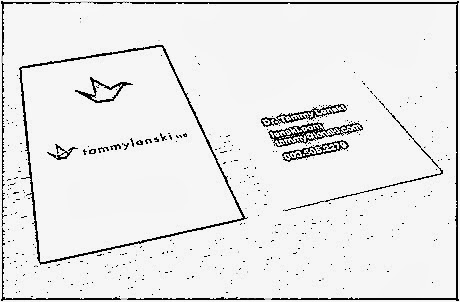graph_03(process by adaptive mean)

Kmeans

As you can see, the results(graph_01~graph_03) can't cut out the second card.Luckily, the colors of the cards and the background are different, a fairly good example for kmeans.

To adopt the cv::kmeans, we need to transfer the image into a samples, each data set of the samples should consist a pixel groups(ex : bgr, rgb, hsv and so on).To generate the image after color segmentation, we have to map the centers generated by the cv::kmeans to the image.

```#include <iostream>

#include <opencv2/core/core.hpp>
#include <opencv2/highgui/highgui.hpp>
#include <opencv2/imgproc/imgproc.hpp>

int main()
{
cv::Mat src = cv::imread(Folder + "perspective05.jpg");
if(src.empty()){
return -1;
}

//step 1 : map the src to the samples
cv::Mat samples(src.total(), 3, CV_32F);
auto samples_ptr = samples.ptr<float>(0);
for( int row = 0; row != src.rows; ++row){
auto src_begin = src.ptr<uchar>(row);
auto src_end = src_begin + src.cols * src.channels();
//auto samples_ptr = samples.ptr<float>(row * src.cols);
while(src_begin != src_end){
samples_ptr = src_begin;
samples_ptr = src_begin;
samples_ptr = src_begin;
samples_ptr += 3; src_begin +=3;
}
}

//step 2 : apply kmeans to find labels and centers
int clusterCount = 3;
cv::Mat labels;
int attempts = 5;
cv::Mat centers;
cv::kmeans(samples, clusterCount, labels,
cv::TermCriteria(CV_TERMCRIT_ITER | CV_TERMCRIT_EPS,
10, 0.01),
attempts, cv::KMEANS_PP_CENTERS, centers);

//step 3 : map the centers to the output
cv::Mat new_image(src.size(), src.type());
for( int row = 0; row != src.rows; ++row){
auto new_image_begin = new_image.ptr<uchar>(row);
auto new_image_end = new_image_begin + new_image.cols * 3;
auto labels_ptr = labels.ptr<int>(row * src.cols);

while(new_image_begin != new_image_end){
int const cluster_idx = *labels_ptr;
auto centers_ptr = centers.ptr<float>(cluster_idx);
new_image_begin = centers_ptr;
new_image_begin = centers_ptr;
new_image_begin = centers_ptr;
new_image_begin += 3; ++labels_ptr;
}
}

cv::Mat binary;
cv::Canny(new_image, binary, 30, 90);

cv::imshow("original", src);
cv::imshow("binary", binary);
cv::imshow( "clustered image", new_image );

cv::waitKey();

return 0;
}
```

In step 1, we rearrange src to sample as following

src :
(b00,g00,r00) (b01,g01,r01)  (b02,g02,r02).......
(b10,g10,r10) (b11,g11,r11)  (b12,g12,r12).......
............

to

sample :
(b00,g00,r00)
(b01,g01,r01)
(b02,g02,r02)
...........
(b10,g10,r10)
(b11,g11,r11)
(b12,g12,r12)
................

The results of the labels are associated with the samples generated by cv::kmeans.The order of reading the labels should be the same as you generated the samples.graph_04(process by kmeans)graph_05(apply canny on graph_04)

Now we could cut the cards from graph_04 without much troubles.To simplify the procedures of kmeans segmentation, I encapsulate it by class.The codes can download from github.

## Saturday, 19 October 2013

### openCV and color quantization--00 : simple algorithm

There are two famous algorithms--pyrMeanShiftFiltering and kmeans could help us quantize the colors of the image.If you want to quantize the color without number of color, pick pyrMeanShiftFiltering, else pick kmeans.

If you don't need the power of kmeans or pyrMeanShiftFiltering, the openCV2 computer vision application programming cookbook introduce a simple algorithm which could reduce the color of the images.I do some refinemet to the codes(use cv::LUT to replace hand made loop).

```#include <iostream>

#include <opencv2/core/core.hpp>
#include <opencv2/imgproc/imgproc.hpp>
#include <opencv2/highgui/highgui.hpp>

void color_reduce(cv::Mat &input, cv::Mat &output, size_t div)
{
if(input.data != output.data){
output.create(input.size(), input.type());
}

uchar buffer;
for(size_t i = 0; i != 256; ++i){
buffer[i] = i / div * div + div / 2;
}
cv::Mat table(1, 256, CV_8U, buffer, sizeof(buffer));
cv::LUT(input, table, output);
}

int main()
{
"/pic/perspective08.jpg");
if (src.empty()){
std::cerr<<"can't open image"<<std::endl;
return - 1;
}

cv::Mat output;
color_reduce(src, output, 64);

cv::imshow("result", output);
cv::imshow("src", src);
cv::waitKey();

return 0;
}
```originalafter quantize

## Friday, 18 October 2013

### perspective correction for quadrilateral markers

When I am studying the marker base augmented reality, I come to realize that the markers in the images always has perspective distortion.To read the information from the markers correctly, we need to correct the distortion.

The link show us how to detect the points of the quadrilateral by cv::HoughLinesP, it works well, but what if there are more than one quadrilateral in the image or there are more than four segments we could detected(graph_00)?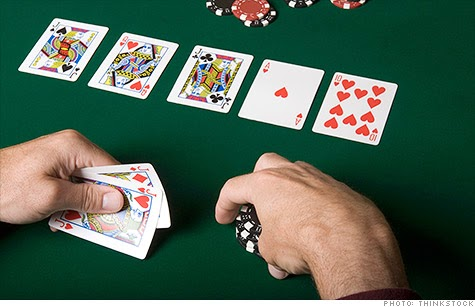graph_00

If we apply cv::HoughLinesP on the graph_00, there will more than 4 lines(graph_01), so the solution suggested by the link can't work on this case.Apparently, we need another approach to reach our goal.

```cv::Mat src = cv::imread("/Users/Qt/program/blogsCodes/"
"pic/perspective08.jpg");
if (src.empty()){
std::cerr<<"can't open image"<<std::endl;
return;
}
cv::Mat bw;
cv::blur(src, bw, cv::Size(3, 3));
cv::Canny(bw, bw, 30, 90);

std::vector<cv::Vec4i> lines;
cv::HoughLinesP(bw, lines, 1, CV_PI / 180, 70, 30, 10);

for(auto const &data : lines){
cv::line(src, cv::Point(data, data),
cv::Point(data, data),
cv::Scalar(0, 0, 255));
}
```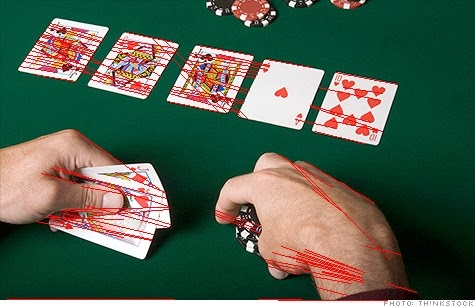graph_01

step 1 : convert the graph_00 to binary image by otsu-threshold

```cv::Mat binary;
cv::cvtColor(src, binary, CV_BGR2GRAY);
cv::threshold(binary, binary, 0, 255, cv::THRESH_BINARY + cv::THRESH_OTSU);
```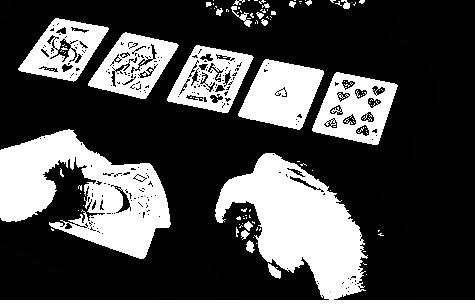graph_02

step 2 : find the contours of binary image

```ContourType find_contours(cv::Mat const &binary_input)
{
ContourType contours;
cv::findContours(binary_input, contours,
CV_RETR_EXTERNAL, CV_CHAIN_APPROX_NONE);

return contours;
}
//.....
auto contours = find_contours(binary);
```

step 3 : find the corners of quadrilaterals(pokers on the table)

```CornersType get_corners(ContourType &contours)
{
//remove the contours size < 100 and size > 100
OCV::remove_contours(contours, 100, 50000);

std::vector approx;
CornersType corners;
for(auto const &data : contours){
cv::approxPolyDP(data, approx,
cv::arcLength(data, true) * 0.02, true);
if(approx.size() == 4 && cv::isContourConvex(approx)){
OCV::sort_rect_corners(approx);
corners.emplace_back(std::move(approx));
}
}

return corners;
}
//.....
auto const corners = get_corners(contours);
```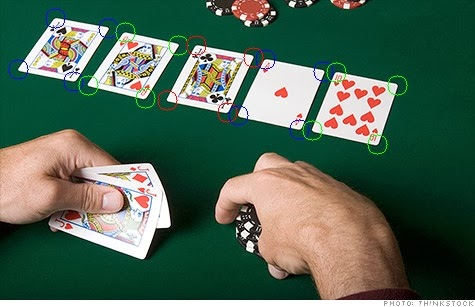graph_03
To get the corners, we need to

1 : remove small contours
2 : approximate the contours to a polygon.
3 : the polygon should have 4 points.
4 : it should be a convex
5 : sort the corners by the order of top left, top right, bottom right, bottom left

check github to get the implementation of OCV::sort_rect_corners.The implementation of OCV::sort_rect_corners is base on the link but with some refinement.

step 4 : correct the perspective distortion by cv::warpPerspective

```cv::Mat target(150, 150, src.type());
std::vector<cv::point2f> target_points
{
{0, 0}, {target.cols - 1, 0},
{target.cols - 1, target.rows - 1},
{0, target.rows - 1}
};
std::vector points;
for(size_t i = 0; i != corners.size(); ++i){
for(auto const &point : src){
points.emplace_back(point.x, point.y);
}
cv::Mat const trans_mat = cv::getPerspectiveTransform(points,
target_points);
cv::warpPerspective(src, target, trans_mat, target.size());
}
```

To project the original poker, we need to declare a new image and two group of points.The first group is the corners point of the original image, the second group is the region we want to project to.In most of the times we would set the points of the second group as large as the new image.Do remember to keep the order of the two group of points same, else the direction of the poker after projection will vary.graph_04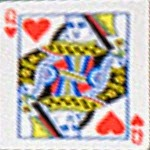graph_05graph_06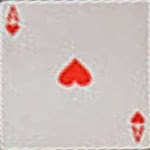graph_07graph_08

The codes could download from github. The lib used by this small project could be found at here. Do not need to build the whole library, what you need to do is include the header files and compile the utility.cpp with your project.

## Saturday, 12 October 2013

c++11 offer us a thread library with ease of use api(although it still lack decent thread pool and high level algorithms like intel TBB or QtConcurrent provided), but I always confused with std::async, std::package_task and std::promise, so I write down this post to help me memorize the different between them.

std::async

we could spawn a thread by std::thread, the api of std::thread is simple and clean, but what if we want to take back the result after process?std::thread do not give us a direction way to do that, this is the time when std::async comes in.

std::async is a high level api for us to start an asynchronous task when we don't need to take the result back right away.

```#include <algorithm>
#include <future>
#include <iostream>
#include <iterator>
#include <vector>

inline int accumulate(std::vector<int> const &data)
{
return std::accumulate(std::begin(data), std::end(data), 0);
}

struct Accmulate
{
int operator()(std::vector<int> const &data)
{
return std::accumulate(std::begin(data), std::end(data), 0);
}
};

int main()
{
std::vector<int> data(100);
std::iota(std::begin(data), std::end(data));

//called by function
std::future<int> result = std::async(accumulate, std:cref(data));
std::cout<<result.get()<<std::endl; //#1

//called by functor
Accmulate acc;
std::future<int> result2 = std::async(&Accmulate::operator(),
&acc, std::cref(data));
std::cout<<result2.get()<<std::endl; //#2
}
```

At #1 and #2 the program will wait until the function and the functor finish the tasks.There are three additional flag for std::async, they are std::launch::async and std::launch::deferred.

• case a : if the std::launch::async is set, the std::async will execute the function or functor in a new thread as if spawned by std::thread.
• case b : if the std::launch::deferred is set, the function call will be deferred until wait() or get() is called by the future.
• By default, the implementation will choose which case should be picked, either case a or case b.

What if we need more sophisticated control about std::async, like saving a lot of "tasks", defer the execution of those asynchronous or concurrent tasks?This is what std::package_task good for.

```#include <future>
#include <iostream>
#include <vector>

inline int max(int a, int b)
{
return std::max(a, b);
}

int main()
{
std::vector<std::future<int>> futures;
for(size_t i = 0; i != 4; ++i){
}

for(auto &data : futures){
std::cout<<data.get()<<std::endl;
}
```

The codes seem no harms at first, but what if exception thrown?Well, boost::scoped_thread(this should be part of the standard) could help us guard the thread and make sure it will call "join()" when leaving the scope.

std::promise

The promise is a building block to communicate with future.We could build std::async by std::package_task;build std::package_task by std::promise.

```// promise example
#include <iostream>
#include <functional>
#include <future>

void test(std::future<int>& input) {
std::cout << fut.get() << std::endl;
}

int main ()
{
std::promise<int> pm;

std::future<int> result = pm.get_future();

pm.set_value (10); #3

th1.join();
return 0;
}
```

we set the value of promise and #3(fulfilled the promise), if we do not set the value before of promise and let the promise exit in this case, the program will throw exception(we can set the exception promise would throw by set_exception()).We cannot

• case 1 : call set_value() of a same promise more than one time
• case 2 : call get_value() of a same promise more than one time
• case 3 : If the promise die without consequence(call set_value()) and the users call the get() of corresponding future, exception will throw.
• case 4 : promise can die without consequence, but the users must not call the get() of corresponding future
case 1 :
```void test()
{
std::promise<int> pm;
pm.set_value(10);
pm.set_value(10); //throw exception
}
```

case 2 :
```void test()
{
std::promise<int> pm;
std::future<int> ft1 = pm.get_future();
std::future<int> ft2 = pm.get_future(); //throw exception
}
```

case 3 :
```void test()
{
std::future<int> ft;
{
std::promise<int> pm;
ft = pm.get_future();
}
ft.get(); //throw exception
}
```

case 4 :
```void test()
{
std::future<int> ft;
{
std::promise<int> pm;
ft = pm.get_future();
}
//ft.get(); if you don't call it, the exception wouldn't thrown
}
```

## Wednesday, 9 October 2013

### Subpixel accuracy--00 : what is subpixel?

In the world of digital image, the smallest unit(information) you can get is pixel, it is impossible for you to access the information between pixels(sub-pixel).Fortunately, with some simple mathematical tricks, we can get over this problem.

Why sub-pixel matter?

Because sometimes we need more accuracy than the original image can provide.

ex :
• Camera calibration(ex : measure homography matrix)
• Stereo matching
• Tracking(ex : SIFT use it to measure feature point)

How to find subpixelgraph_00(from wikepedia)

A graph is worth a thousand words. With the help of bilinear interpolation, we could measure the subpixel at ease. Our target is the value of the pixel located at (20.2, 14.5).As you see, the coordinate of the target is fractional but not integer.

Equation (1), (2) and (3) show you how to measure the value of the target. If you need more details, please check out the wiki.

Implement by codes

```float get_sub_pix(cv::Mat const &img, cv::Point2f const &pt)
{
int x = static_cast<int>(pt.x);
int y = static_cast<int>(pt.y);

int x0 = cv::borderInterpolate(x,   img.cols, cv::BORDER_REPLICATE);
int x1 = cv::borderInterpolate(x+1, img.cols, cv::BORDER_REPLICATE);
int y0 = cv::borderInterpolate(y,   img.rows, cv::BORDER_REPLICATE);
int y1 = cv::borderInterpolate(y+1, img.rows, cv::BORDER_REPLICATE);

float a = pt.x - x;
float c = pt.y - y;

float x1_interpolate = (img.at<uchar>(y0, x0) * (1.0 - a)
+ img.at<uchar>(y0, x1) * a);
float x2_interpolate = (img.at<uchar>(y1, x0) * (1.0 - a)
+ img.at<uchar>(y1, x1) * a);
float target = x1_interpolate * (1.0 - c) + x2_interpolate * c;

return target;
}
```

There are more than one way to find the subpixel, this is only one of them.As usual, the codes can download from github.

## Monday, 7 October 2013

### Harris Corners--01 : implement by openCV2

In this post I would like to analyze the Harris corners of openCV2, original source codes are located at github of openCV.To make it easier to read, I do some change to the source codes.

Codes

```void harris_corners(cv::Mat const &input, cv::Mat &output,
int block_size, int ksize, float k)
{
//step 1 : make sure the type of the input is valid
if(!(input.type() == CV_8U || input.type() == CV_32F)){
throw std::runtime_error(COMMON_DEBUG_MESSAGE +
"input.type() != CV_8U ||"
" input.type() != CV_32F\n");
}

output.create(input.size(), CV_32F);

//step 2 : scale need to normalize the result of the sobel filter
//I don't get it why they initialize the scale like this
//if anyone figure it out please tell me why, thanks
double scale = (double)(1 << ((ksize > 0 ? ksize : 3) - 1))
* block_size;

//the range of CV_8U are in range [0, 255];
//CV_32F are in range [0, 1]
if(input.type() == CV_8U){
scale *= 255.0;
}
scale = 1.0/scale;

//step 3 : calculate the gradient of x and y direction
cv::Mat dx, dy;
cv::Sobel(input, dx, CV_32F, 1, 0, ksize, scale);
cv::Sobel(input, dy, CV_32F, 0, 1, ksize, scale);

cv::Size size = input.size();
//step 4 : convolution and save dx*dx, dx*dy, dy*dy in cov
using Type = float;
cv::Mat cov(size, CV_32FC3);
for(int i = 0; i < size.height; ++i){
Type *cov_data = cov.ptr<Type>(i);
Type const *dxdata = dx.ptr<Type>(i);
Type const *dydata = dy.ptr<Type>(i);

for(int j = 0; j < size.width; ++j){
Type const dx = dxdata[j];
Type const dy = dydata[j];
*cov_data = dx*dx; ++cov_data;
*cov_data = dx*dy; ++cov_data;
*cov_data = dy*dy; ++cov_data;
}
}

//step 5 : generate the result of Harris matrix,
//all of the weight are set as 1
cv::boxFilter(cov, cov, cov.depth(), cv::Size(block_size, block_size),
cv::Point(-1,-1), false);

//step 6 : optimize the iteration speed, not a neccessary
//step for harris_corner
if( cov.isContinuous() && output.isContinuous() ){
size.width = input.total();
size.height = 1;
}

//step 7 : find out Mc and save it to the output Mat
for(int i = 0; i < size.height; ++i){
Type const *cov_data = cov.ptr<Type>(i);
Type *dst = output.ptr<Type>(i);
for(int j = 0; j < size.width; ++j){
Type const a = *cov_data; ++cov_data;
Type const b = *cov_data; ++cov_data;
Type const c = *cov_data; ++cov_data;
Type const temp = a + c;
dst[j] = a*c - b*b - k*(temp)*(temp);
}
}
}
```

The most curious step is step 2, I have no idea why are they select a scale like that?If anybody know the answer, please give me some comment or leave the answer at openCV forum.

Resultsgraph_00graph_01

Shi-Tomashi improve the result of Harris corners, openCV2 implemented it as function "cv::goodFeaturesToTrack".

## Saturday, 5 October 2013

### Harris Corners--00 : principle

Harris Corner is an famous algorithm to locate the useful features(corners) in the image.Now there are two questions for us to understand.

1 : What are features
• Exactly, there are no formal definition of feature yet(atleast I can't find it), in the world of computer vision, you could treat it as the information you use for tracking, discern the objects(just my intuition, if you find any errors, please give me some comments).

2 : Why choose corners as features?
• Because in many cases, corners are good features.They provide enough messages for the program to discern the location of the objects.

3 : How do we find the corners?
This is the main part of this post, now let me try to explain it.

In a nutshell, we use the lambdas of Hessian Matrix(1) to measure the change rate of the vectors.There are three conditions

1. lambda one and lambda two are small, that means this pixel belong to a flat region.
2. lambda one or lambda two are big, that means this pixel belong to edges.
3. lambda one and lambda two are big, that means this pixel belong to corners.

So far,so good, but why do this work? From graph_00, we discovered that the region around the corners are changing rapidly, that means if the pixel belong the the corner, the pixels surrounded it have a high chance to change rapidly for every direction.graph_00

Computation of the lambdas are very expensive, so Harris and Stephens proposed a function Mc(2) to approximate the it.

graph_01(I take it from the original paper) explain what are the Mc doing.We could decide which Mc qualify the condition as corner.graph_01

4 : Where are the Hessian matrix come from?

The Hessian Matrix are deduced from the sum of square difference(3), (4) is approximated by the taylor series(only take the zero and first derivative terms).Substitude (4) to (3), we get (5), and we can express (5) to the new equation--(6). The A of (6) is the Harris matrix we care about.